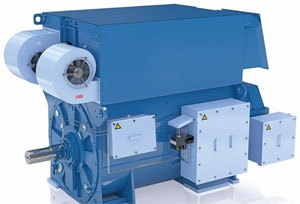Categories

# How to calculate the power losses of Generator Set?

The purpose of a generator set is to transform the energy in the fuel used by the prime mover into electrical energy at the generator terminals. Since nothing is perfect, the amount of energy input is ALWAYS greater than the amount of energy output, resulting in an efficiency that is ALWAYS less than 100 percent.

Every fuel has a basic "heat value": this is usually broken down into two cases. The first case uses a LOW value that corresponds to the assumption where the water vapor created during combustion remains gaseous, ensuring that the latent heat of vaporization on that amount of water is not available. The second case is a HIGH value, assuming that the water condenses and the latent heat is available. In reality, there's some of both going on at any given moment. This means that the LOW case is somewhat conservative in its estimation, and the HIGH case is overly optimistic.So - every fuel-powered prime mover has some sort of BFSC (brake specific fuel consumption) rating. Testing by the prime mover manufacturer produces a set of curves for a given design, which shows the fuel consumption to produce a specific amount of mechanical output power. This mechanical power is then the input to the electrical machine, which then converts the mechanical input into electrical output. Along the way, there are losses: the waste heat from fuel combustion, the mechanical losses of the prime mover components, windage and friction in the prime mover, drive train (coupling and/or gearing) mechanical losses, drive train windage and friction, drive train waste heat (for gearboxes, at least), electrical machine windage and friction, electrical machine mechanical losses, electrical machine waste heat, electrical machine electrical losses (I2R), and electrical machine magnetic losses (core loss).

Typically, the prime mover has an "efficiency" for a given operating point, as does any mechanical structure in the drive train - such as a gearbox - and the electrical machine also has an efficiency rating. To obtain the total efficiency for the generator set, multiply the component efficiencies together. This means the calculation:
(SET EFF) = (PRIME EFF) * (TRAIN EFF) * (GEN EFF)

which in turn means that the set efficiency is always going to be lower than that of any individual component, since all efficiencies are less than unity.

Once you have the "set" efficiency and a required output of electrical energy, work backward to determine the necessary input mechanical power and therefore the amount of fuel consumption.

Note that different fuels have different energy content and thermal profiles and will thus have an impact on the BFSC. Environmental conditions such as temperature, humidity, and altitude will also necessitate an "adjustment" to the equation.

Generator , How to